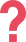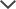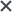Note: the SBAC Practice Pack is NOT included in our Premium Membership.

The SBAC Summative Assessments for 3rd–5th grade are year-end tasks that track how students are progressing toward their academic goals. SBAC results are often used to determine student eligibility into accelerated programs in elementary school years. SBAC Tests are aligned with the Common Core. This means that every component of the SBAC for 3rd, 4th, or 5th grade is based on content claims and assessment targets that correspond with Core Curriculum standards. Like all the SBAC Tests, SBAC for 3rd, 4th, and 5th grade assesses students in both English Language Arts (ELA) and Mathematics.

## SBAC English Language Arts (ELA)

The ELA Test for 3rd, 4th, and 5th grades is comprised of two types of exams: the performance task (PT) and the computer adaptive test (CAT). Both tests are designed using content claims that have been predetermined by the SBAC Board of Education. The content claims for the ELA Test cover the following topics:

• Writing
• Listening and speaking
• Research and inquiry

The ELA performance task provides students with two short texts that they must research and use as reference to answer a series of questions. Additionally, students must complete one writing task that demonstrates their grasp of proper writing organization, proper writing conventions and appropriate evidence usage. Students are given two hours to complete the performance task.

The ELA CAT is an untimed computerized test that increases or decreases in difficulty depending on student response. Students are tested using multiple-choice questions or short answer questions that assess them across all four content claim areas. For more information about the SBAC ELA Test, and for even more SBAC practice online, visit our SBAC ELA page.

## SBAC Math for 3rd Grade

The SBAC Math Test for 3rd grade is comprised of a performance task exam and a computer adaptive test. The performance task is made up of 4–6 items that require multi-step problem-solving. These items take students, on average, two hours to complete. The CAT section is untimed and includes a series of question types testing students across a range of mathematical skills in alignment with a standard 3rd grade math level.

The SBAC Math Test has its own set of predetermined content claims that require students to apply mathematical concepts, problem solve, communicate reasoning, and use modeling and data analysis. Questions on the SBAC Math Test for 3rd grade cover the following areas:

• Operations and algebraic thinking
• Number and operations utilizing ten as a base
• Number and operations utilizing fractions
• Geometry

## SBAC Math for 4th and 5th Grade

Like the SBAC for 3rd grade, the SBAC Math Test for 4th and 5th grade is designed around four main content claims. These claims are meant to assess student mathematical ability in accordance with a standard 4th or 5th grade curriculum.

The performance task section provides students with a multi-step,real-lifee scenario and asks them to solve the problem at hand using mathematical skills and logic. This task takes students up to two hours to complete.

The CAT section is a computerized test providing students with a series of multiple-choice questions. These questions assess students in the following mathematical areas:

• Operations and algebraic thinking
• Number and operations utilizing ten as a base
• Number and operations utilizing fractions
• Geometry
• Measurement and data

The SBAC Math Test for 5th grade is designed using the exact same breakdown of test items. However, the questions are more advanced and ask students to utilize a higher level of mathematical abilities. For example, whereas the 4th grade geometry component of the test requires students to identify and draw lines and angles, or to classify shapes by certain properties, in 5th grade, geometry test items may ask students to analyze graph points on a coordinate plane in order to solve real-world or mathematical problems.

For a more detailed explanation of the SBAC Math Test, and for even more SBAC practice, visit our SBAC Math page.

## SBAC Scores for 3rd, 4th, and 5th Grade

SBAC tests are scored using two methods. The first method, the scale score method, provides each student with a numerical value ranging between 2000 and 3000. These scores can be used to compare students within a given class or for national ranking comparisons. The second score method utilized is achievement level scores. These levels range from 1–4 and are determined based on student scale scores. To view a breakdown of achievement levels and scale scores corresponding to grade level, visit the scores section of our SBAC Practice page.

## SBAC Practice Tests for 3rd, 4th, and 5th Grade

Need HelpNeed Help